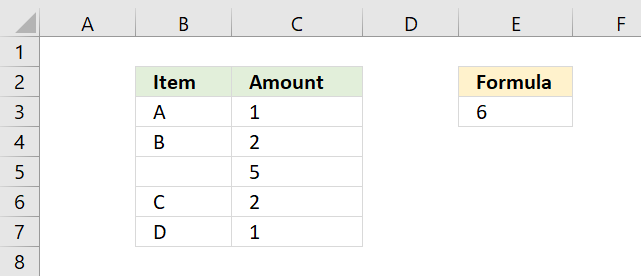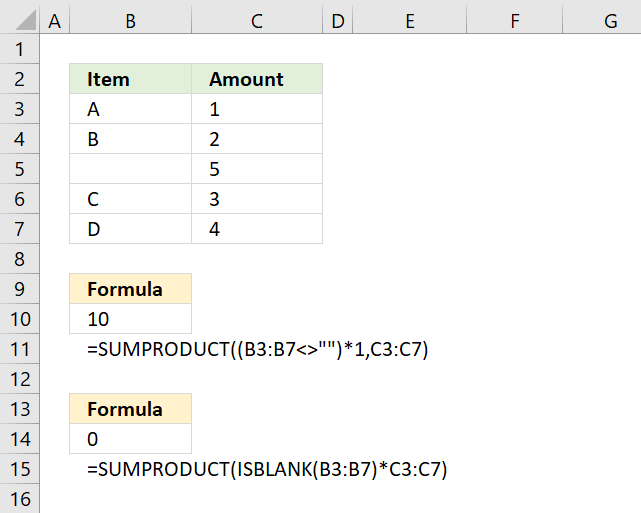Author: Oscar Cronquist Article last updated on February 05, 2018The above image demonstrates how to ignore blank cells in a SUMPRODUCT formula. The following formula is shown in cell E3.

=SUMPRODUCT(NOT(ISBLANK(B3:B7))*C3:C7)

There is no need for an IF function, simply use the ISBLANK function and then multiply with the corresponding cell range.

ISBLANK(B3:B7)

The ISBLANK function returns TRUE or FALSE determined by if cell is blank or not. Since we are using a cell range the ISBLANK function returns an array with the same size as the cell range.

{FALSE;FALSE;TRUE;FALSE;FALSE}

The NOT function comes in handy when you want to convert the boolean values to their opposites. For example, TRUE becomes FALSE and FALSE becomes TRUE.

NOT(ISBLANK(B3:B7))

becomes

{TRUE;TRUE;FALSE;TRUE;TRUE}

The next step is to multiply the boolean array with cell range C3:C7.

NOT(ISBLANK(B3:B7))*C3:C7

becomes

{TRUE;TRUE;FALSE;TRUE;TRUE}*{1;2;5;2;1}

and returns

{1;2;0;2;1}

The SUMPRODUCT function then adds all numerical values in the array returning 6 in cell E3.

SUMPRODUCT({1;2;0;2;1})

1+2+0+2+1 = 6

### Formula returns blankCell range B3:B7 contains a formula that sometimes returns a character and sometimes a blank. The ISBLANK function won't work in this case, see cell B14, it returns 0 which is incorrect.

We need to rely on larger than and smaller than characters <>, see formula in cell B10.

=SUMPRODUCT((B3:B7<>"")*C3:C7)

Together like this <> means not equal to. Two double quotes "" is nothing.

B3:B7<>"" is a logical expression and returns an array of boolean values with as many values as the number of cells in the cell range B3:B7.

{TRUE;TRUE;FALSE;TRUE;TRUE}

The parentheses determine the order of calculations, we need it to compare the cell range with nothing before multiplying with the cell range.

(B3:B7<>"")*C3:C7

becomes

{TRUE;TRUE;FALSE;TRUE;TRUE}*C3:C7

becomes

{TRUE;TRUE;FALSE;TRUE;TRUE}*{1;2;5;3;4}

and returns

{1;2;0;3;4}

The SUMPRODUCT function sums all values in the array.

SUMPRODUCT({1;2;0;3;4})

and returns 10 in cell B10. 1+2+0+3+4 = 10.